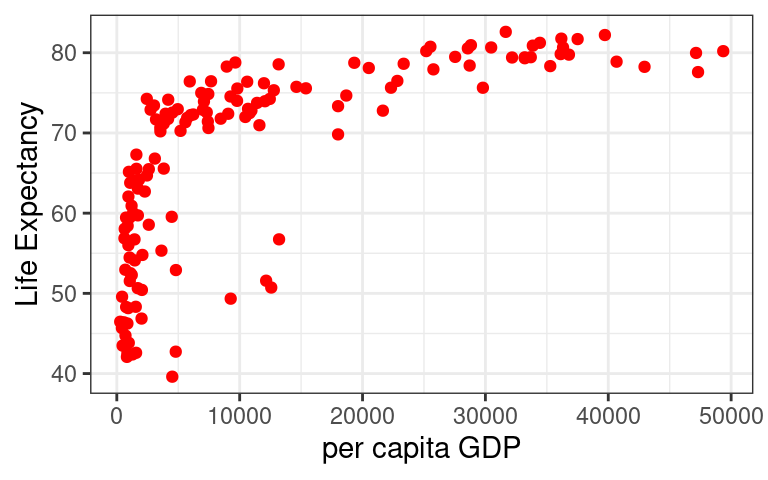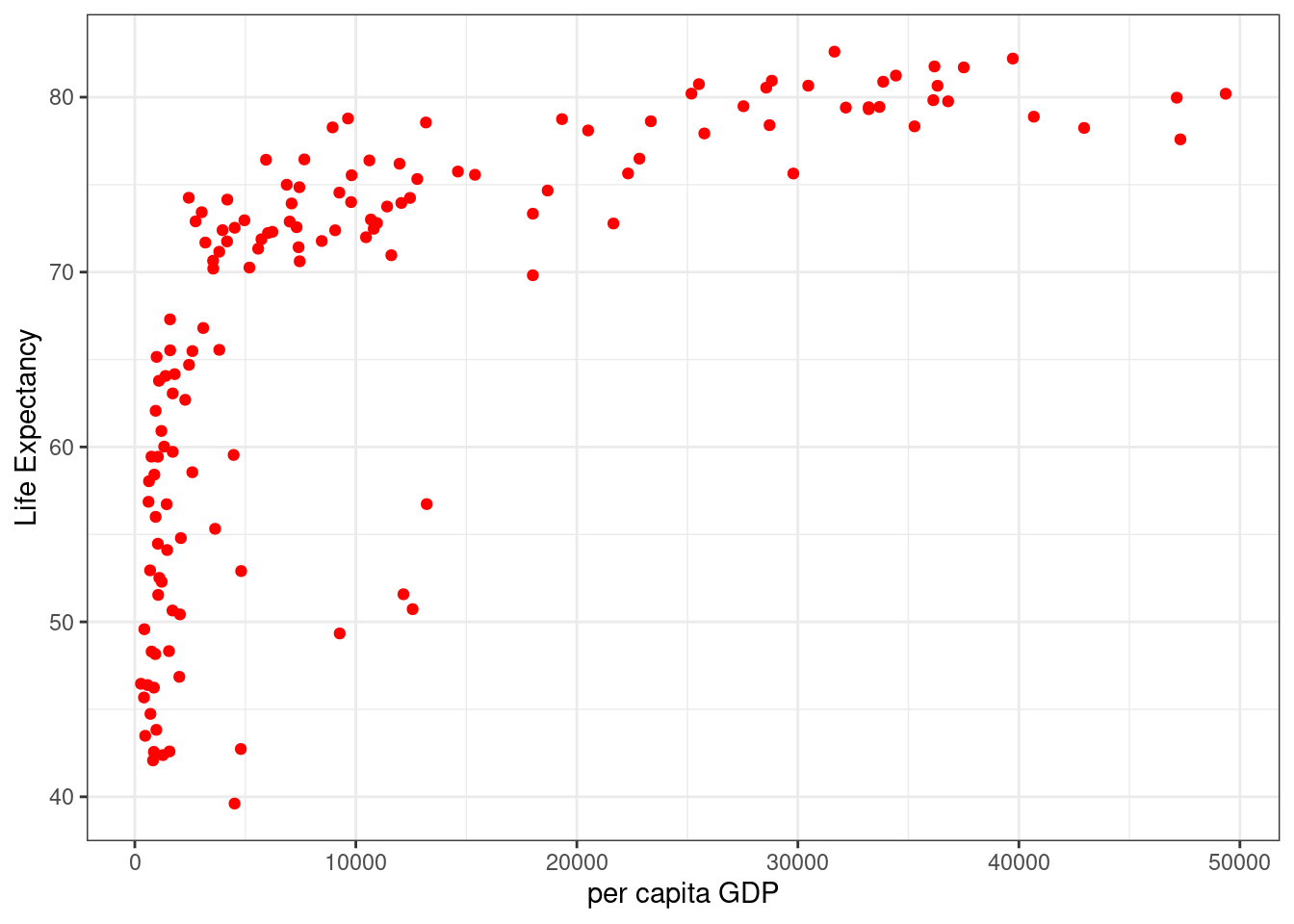For today’s code challenge, you will need to create a plot of the relationship between a country’s per capita GDP and life expectancy. To start with, install the gapminder package. Once you call library(gapminder), this will give you a data.frame called gapminder. Using data only from 2007, I’d like for you to exactly recreate the following plot using the ggplot2 package:Rules:

• You cannot load any external packages (besides ggplot2 and gapminder), but any base R functions are allowed

To win

• You must email me your code brantly.callaway@uga.edu

• I’ll run exactly the code that you send me, and if it can exactly reproduce the plot, then you win.

Solution below…

library(gapminder)
library(ggplot2)

gm2007 <- subset(gapminder, year==2007)
ggplot(data=gm2007,
mapping=aes(x=gdpPercap, y=lifeExp)) +
geom_point(color="red") +
ylab("Life Expectancy") +
xlab("per capita GDP") +
theme_bw()